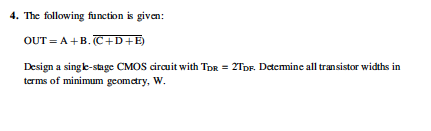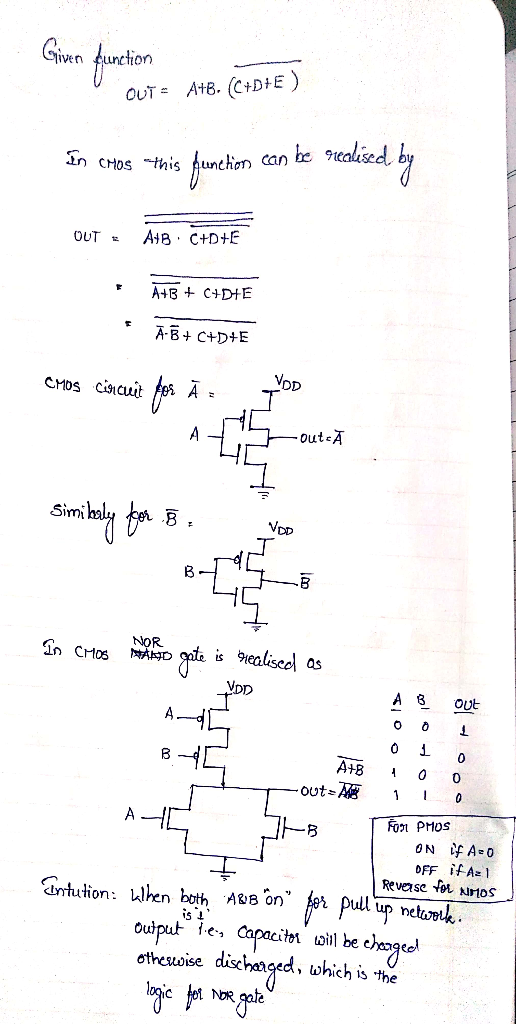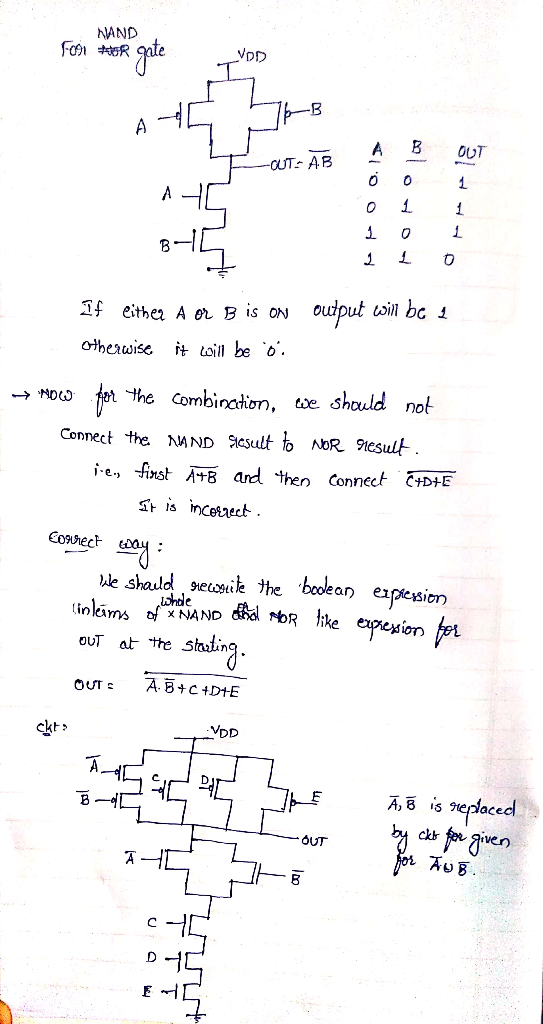# 4. The following function is given: OUT = A + B. (C + D + E) Design a singk-stage CMOS circuit wi...I'm new to the subject. Please explain the steps where possible.

4. The following function is given: OUT = A + B. (C + D + E) Design a singk-stage CMOS circuit with Tor 2TDF Determine all transistor widths in terms of minimum geometry, W##### Add Answer of: 4. The following function is given: OUT = A + B. (C + D + E) Design a singk-stage CMOS circuit wi...
More Homework Help Questions Additional questions in this topic.

• #### 1.5.5 In Class Exercise: Work out the following examples from the text. Design CMOS logic functio...

Need Online Homework Help?